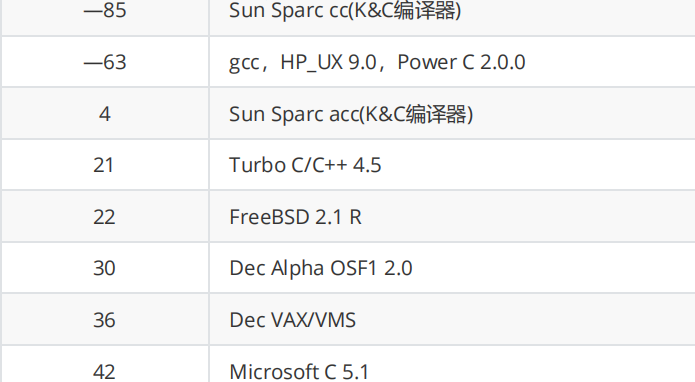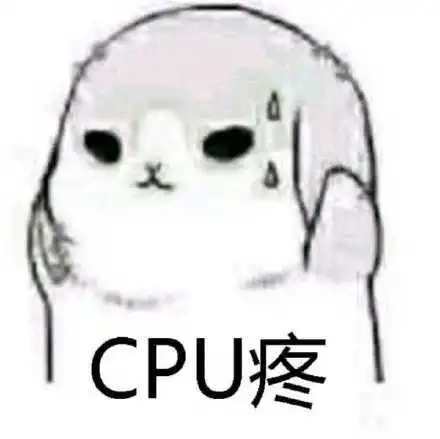# C语言操作符大全(建议收藏)

💖作者龟龟不断向前

👻专栏C++初阶知识点

Schedule

👻工具分享

MySQL读写分离

1. 刷题： 牛客网 leetcode
2. 笔记软件：有道云笔记
3. 画图软件：Xmind(思维导图) diagrams(流程图)try

## C语言操作符

### 1.操作符分类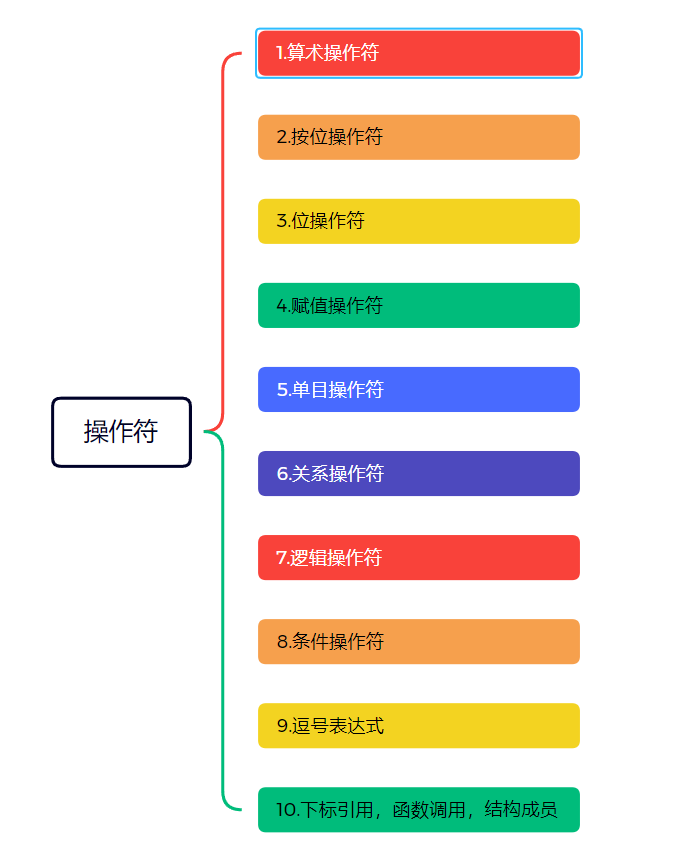### 2.算术操作符

``````+    -   *   /   %
``````

1. 除了 `%` 操作符之外，其他的几个操作符可以作用于整数和浮点数。

WSA

2. 对于 `/` 操作符如果两个操作数都为整数，执行整数除法。而只要有浮点数执行的就是浮点数除法

cout

``````#include<stdio.h>

int main()
{
printf("%d\n", 3 / 2);//整数除法
printf("%.1f\n", 3.0 / 2);//浮点数除法
}
``````

3. `%` 操作符的两个操作数必须为整数。返回的是整除之后的余数。

spartan-3E

#### 1.1%的应用 – 求解最大公因数

FreeMarker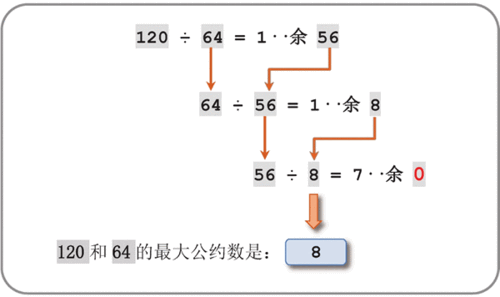``````#include<stdio.h>

int max_common_fac(int m, int n)
{
int r = 0;
do
{
r = m % n;
m = n;//m取上一次的除数
n = r;//n取上一次的余数
} while (r);//直到r为0，此时的除数为最大公因数

return m;
}

int main()
{
int m = 0, n = 0;
scanf("%d %d", &m, &n);

int ret = max_common_fac(m, n);
printf("%d和%d的最大公因数为%d\n", m, n ,ret);
return 0;
}
``````

#### 1.2%的应用 – 拆出一个数的每一位

``````#include<stdio.h>

//为了让效果明显一点，咱们将每一位打印出来
void seq_print(int n)
{
if (n > 9)
{
seq_print(n / 10);
}

printf("%d ", n % 10);
return;
}

int main()
{
int n = 0;
scanf("%d", &n);

seq_print(n);
return 0;
}
``````

### 3.移位操作符

``````<< 左移操作符
>> 右移操作符
``````

1. 将一个数切换成二进制
2. 再进行移位

HashMap源码

#### 2.1左移

LSTNet

HashMap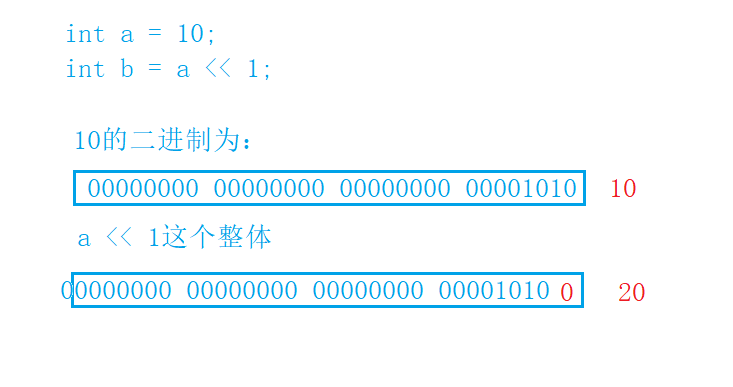ps:是b接收了`a<<1`的值，b为20，但是a还是原来的10，没有变化

#### 2.2右移

1. 逻辑移位

1. 算术移位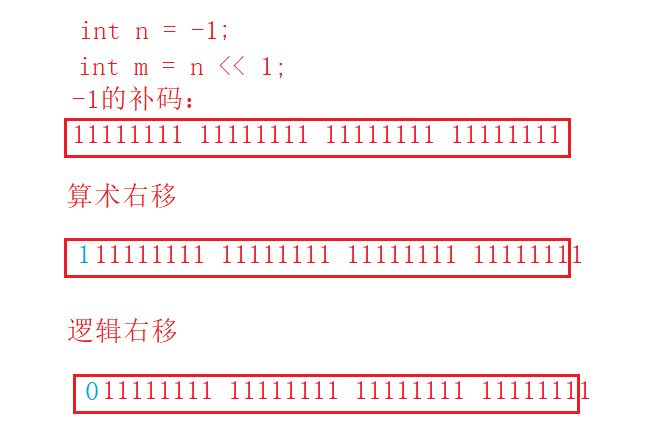ps1:咱们vs编译器的右移使用的是算术右移

### 4.位操作符

``````& //按位与 -- 同1为1，否则为0
| //按位或 -- 同0为0，反则为1
^ //按位异或 -- 相同为0，相异为1

``````

ps:位操作符，相对于二进制位的操作

``````#include<stdio.h>

int main()
{
int a = 3;
//00000000000000000000000000000011 -- 3的补码
int b = -5;
//10000000000000000000000000000101
//11111111111111111111111111111010
//11111111111111111111111111111011 -- -5的补码

//a&b
//00000000000000000000000000000011 -- &之后的结构,符号位是0，原反补一致

//a|b
//11111111111111111111111111111011 -- |之后的结果，此时还是补码，打印出来要转换成原码
//10000000000000000000000000000100
//10000000000000000000000000000101 -- -5

//a^b
//11111111111111111111111111111000 -- ^之后的结果，此时还是补码，打印出来要转换成原码
//10000000000000000000000000000111
//10000000000000000000000000001000  -- -8

printf("a & b = %d\n", a & b);
printf("a | b = %d\n", a | b);
printf("a ^ b = %d\n", a ^ b);
return 0;
}
``````

#### 4.1&与>>的应用 – 计算出二进制下1的个数

1. 通过&1，我们可以得到二进制中的最低位

2. 再配合右移(>>)，即可将二进位的每一位都得到

``````#include<stdio.h>

int main()
{
int a = 0;
while ((scanf("%d", &a)) != EOF)//多组测试用例，方便测试
{
int count = 0;

for (int i = 0; i < 32; ++i)
{
if ((a >> i) & 1)
{
++count;
}
}
printf("%d的二进制的1的个数：%d\n", a, count);
}
return 0;
}
``````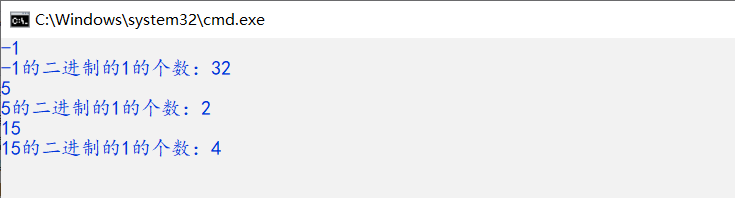#### 4.2^的应用 – 不创建临时变量，交换两个数

0 ^ a = a

a ^ a = 0

a ^ a ^ b = 0

b ^ b ^ a = 0

a ^ b = b ^ a

a ^ b ^ a = b

a ^ b ^ b = a

``````#include<stdio.h>

int main()
{
int a = 3;
int b = 5;
a = a^b;//a此时变成密匙
b = a^b;//密匙 ^ b = a   b此时变成a
a = a^b;//密匙 ^ a = b   a此时变成b

printf("%d %d\n", a, b);
return 0;
}
``````

### 5.赋值运算符

= – 将右操作数赋给做操作数

``````int a = 10;
int x = 0;
int y = 20;
//也可以连续赋值
a = x = y+1;//从右向左赋值
//效果等价于
x = y+1;
a = x;
``````

``````+=
+=效果举例：
int x = 10;
x = x+10;
等价于：
x += 10;//复合赋值
-=
*=
/=
%=
>>=
<<=
&=
|=
^=
``````

### 6.单目操作符

``````!           逻辑反操作
-           负值
+           正值 -- +a -- 对a不做任何处理
&           取地址
sizeof      操作数的类型长度（以字节为单位）
~           对一个数的二进制按位取反
--          前置、后置--
++          前置、后置++
*           间接访问操作符(解引用操作符)
(类型)       强制类型转换
``````

``````#include<stdio.h>

int main()
{
int a = 0;
int b = 7;
printf("!%d = %d\n", a, !a);
printf("!%d = %d\n", b, !b);

printf("&a = %p\n", &a);
printf("&b = %p\n", &b);

printf("%u\n", sizeof(int));
printf("%u\n", sizeof(a));
printf("%u\n", sizeof a);//true
//printf("%u\n", sizeof int); //error sizeof后面不接括号，不能接类型名,但是可以接变量名

printf("%d\n",~0);//-1 -- 二进制的每一位按位取反
return 0;
}
``````

#### 6.1前置，后置的++ –

``````#include<stdio.h>

int main()
{
int a = 10;
//后置++
int b = a++;//先执行b = a ,再执行a++

printf("%d\n", b);//10

printf("%d\n", a);//11
return 0;
}
``````

``````#include<stdio.h>

int main()
{
int a = 10;
//后置++
int b = ++a;//先执行a++,后执行b = a

printf("%d\n", b);//11

printf("%d\n", a);//11
return 0;
}
``````

#### 6.2sizeof和数组

``````#include <stdio.h>

void test1(int arr[])
{
printf("%d\n", sizeof(arr));//(2)
}

void test2(char ch[])
{
printf("%d\n", sizeof(ch));//(4)
}

int main()
{
int arr = {0};
char ch = {0};
printf("%d\n", sizeof(arr));//(1)
printf("%d\n", sizeof(ch));//(3)
test1(arr);
test2(ch);
return 0;
}

//(1) -- 40
//(2) -- 10
//(3) -- 4/8
//(4) -- 4/8
``````

### 7.关系操作符

``````>
>=
<
<=
!=   	用于测试“不相等”
==      用于测试“相等” -- 注意是两个等号
``````

### 8.逻辑操作符

``````&&     逻辑与
||     逻辑或
``````

&& 和 ||的理解有很多种

1. 有些同学理解成botheither
2. 有些同学理解成并且/都或者
3. 有些同学理解成电路中的串联并联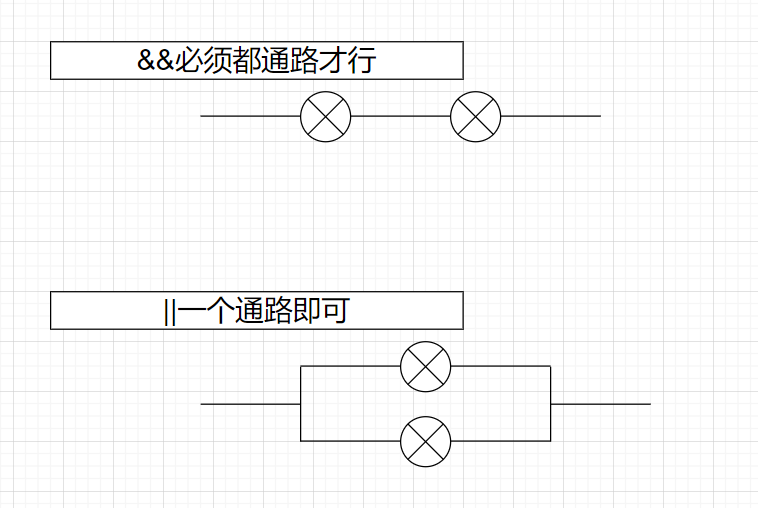``````#include<stdio.h>

int main()
{
int a = 1;
int b = 2;
if (a && b)
{
printf("你们两都来啦\n");
}
else
{
printf("你们两怎么没都来");
}
return 0;
}
``````
``````int main()
{
int a = 0;
int b = 0;
if (a || b)
{
printf("你们两至少有一个来了\n");
}
else
{
printf("你们两怎么没有一个人来\n");
}
return 0;
}
``````

``````

int main()
{
int age = 0;
printf("请输入你的年龄\n");
scanf("%d", &age);
//if (18 <= age <= 36)//逻辑相差很大
if (age >= 18 && age <= 36)
{
printf("你是青年\n");
}
else
{
printf("你不是青年\n");
}
return 0;
}
``````

#### 8.1&& 与 ||的短路问题

360笔试题

``````#include <stdio.h>
int main()
{
int i = 0,a=0,b=2,c =3,d=4;
i = a++ && ++b && d++;
//i = a++||++b||d++;
printf("a = %d\n b = %d\n c = %d\nd = %d\n", a, b, c, d);
return 0;
}
``````

``````#include <stdio.h>
int main()
{
int i = 1,a=0,b=2,c =3,d=4;
i = a++||++b||d++;
printf("a = %d\n b = %d\n c = %d\nd = %d\n", a, b, c, d);
return 0;
}
``````

&&：当遇到假时，后面的表达式便不再执行

||：当遇到真时，后面的表达式便不再执行

### 9.条件操作符

​ 下面的图片可以大致解释条件表达式的意思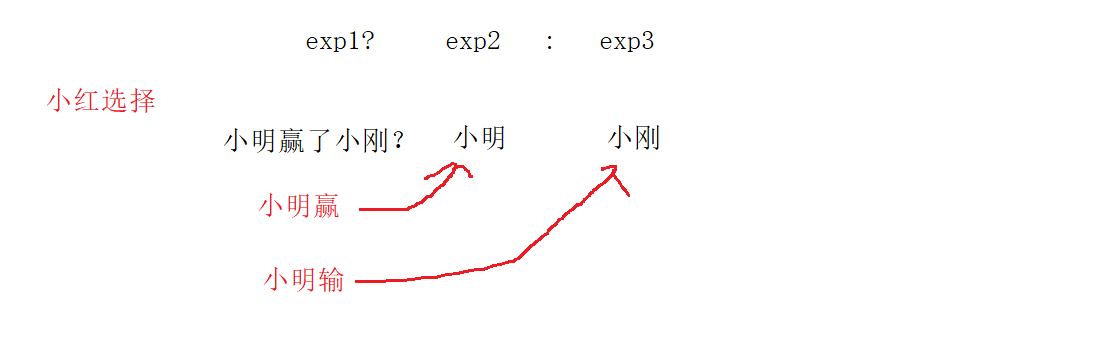``````#include<stdio.h>

int main()
{
int a = 3;
int b = 10;
int c = a > b ? a : b;
printf("c = %d\n", c);
return 0;
}
``````

`exp1?exp2:exp3`，如果`exp1`表达式的值为真，那么整个表达式的值就是`exp2`的值，否则整个表达式的值就是`exp3`的值

``````int Max(int x, int y)
{
if (x > y)
{
return x;
}
else
{
return y;
}
}
``````

``````int Max(int x, int y)
{
return x > y ? x : y;
}
``````

### 10.逗号表达式

``````exp1, exp2, exp3, …expN
``````

ps:由于逗号表达式是所有表达式中，优先级最低的，咱们尽量加上括号

``````(exp1, exp2, exp3, …expN)
``````
``````#include<stdio.h>

int main()
{
int a = 1;
int b = 2;
int c = (a>b, a = b + 10, a, b = a + 1);
printf("%d\n", c);
return 0;
}
``````

###11下标引用、函数调用和结构成员

• `[]` – 下标引用操作符

语法：数组名 + [下标]

``````#include<stdio.h>

int main()
{
int arr;//创建数组
arr = 10;//实用下标引用操作符。
//[ ]的两个操作数是arr和9。
printf("%d\n",arr);
return 0;
}
``````

• `()` – 函数调用操作符

语法： 函数名 + (n个函数参数)

``````#include <stdio.h>
void test1()
{
printf("你好\n");
}

void test2(const char *str)
{
printf("%s\n", str);
}

int main()
{
test1();            //实用（）作为函数调用操作符。
test2("hello world");//实用（）作为函数调用操作符。
return 0;
}
``````

• 访问一个结构的成员

`.`结构体.成员名

`->` 结构体指针->成员名

``````#include <stdio.h>
struct Stu
{
char name;
int age;
char sex;
double score;
};

void set_age1(struct Stu stu)
{
stu.age = 18;
}

void set_age2(struct Stu* pStu)
{
pStu->age = 18;//结构成员访问
}

int main()
{
struct Stu stu;
struct Stu* pStu = &stu;//结构成员访问

stu.age = 20;//结构成员访问
set_age1(stu);//值传递

pStu->age = 20;//结构成员访问
set_age2(pStu);//址传递
return 0;
}
``````

### 12.操作符的属性

1. 操作符的优先级
2. 操作符的结合性
3. 是否控制求值顺序。

&& ||的短路会使每次的表达式求值顺序不一样

#### 12.1编译器也无法知晓的表达式求值

ps:即使有了优先级，结合性，控制求值顺序这些属性，并不就意味着所有的表达式求值都有了一致的求法，代码的不规范，还是会造成编译器也不知该如何处理的情况

``````#include<stdio.h>

int main()
{
int i = 10;
i = i-- - --i * ( i = -3 ) * i++ + ++i;
printf("i = %d\n", i);
return 0;
}
``````

《c和指针》这本书中，作者将改代码放在不同的编译器下跑的结果：